### Home > MC1 > Chapter 4 > Lesson 4.1.3 > Problem4-32

4-32.
1. . Each of the diagrams below represents a sequence for an acrobat on a tightrope. Each letter represents the unknown length of a trick. For each part below, figure out how far the acrobat travels during each trick (that is, the length represented by each letter). Show how you know. Homework Help ✎

1. Find x.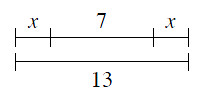2. Find j.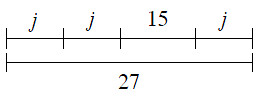3. Find n.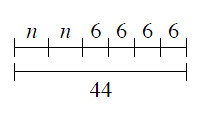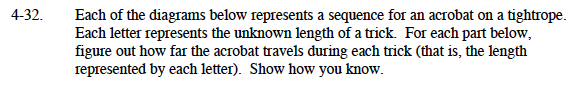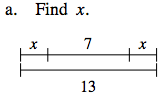If x + 7 + x is the same as 13, can you think of a way to find the value of x?

To find the value of both x's combined, we can subtract 7 from 13.
13 − 7 = 6

Now you know that two x's are equal to 6. Can you find the value of one x?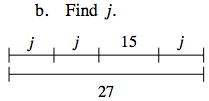Do you think you can use a similar strategy as in part (a)? Remember, try to find the value of just one j.

27 is the same as j + j + 15 + j. Can you find the value of j?

j = 4. Remember to show how you know!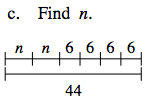Remember that 6 + 6 + 6 + 6 is the same as 6(4). This simplification should make finding n easier.

If two n's equal 20, how much is one n?